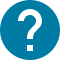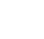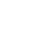大学院
HOME 大学院 農業・資源経済学特別講義Ⅲ (Special Lectures in Agricultural and Resource EconomicsⅢ)

# 農業・資源経済学特別講義Ⅲ (Special Lectures in Agricultural and Resource EconomicsⅢ)

In this course, we learn fundamental mathematics which is frequently used in microeconomics, macroeconomics and econometrics. We use  as a basic textbook. After each lecture, some exercises from the textbook are assigned as homework. The working knowledge you are expected to master through this course will help you to understand the articles related to your thesis work. Since this course is very basic, you do not need to take if you have some knowledge of mathematics. Attendance to the class is not required. Thus, those who have sufficient working knowledge are able to get credit if they register this course and pass the final examination.

コース名

3905531
GAG-AE6E03L2

S1 S2

シラバス シラバス「その他」欄参照

2

NO

Contents Outline 1) Calculus (Differential) 2) Static Optimization (Lagrangean method, Envelope theorem) 3) Matrix Algebra (determinant, system of linear equations, quadratic form, diagonalization) 4) Comparative Statics 5) Introduction to econometrics (OLS, OLS with linear constraint, GLS, ML, GMM) 6) Dynamic Optimization (Calculus of variations, Hamiltonian, Dynamic Programing) 7) Introduction to Differential Equation Many economic applications are introduced.

Lecture is given before exercises.

Evaluation is based on the final examination.

Chiang, Fundamental Methods of Mathematical Economics, McGraw Hill

 Hal Varian, Microeconomic Analysis 3rd ed, Norton  David Romer, Advanced Macroeconomics 4th ed, McGraw Hill  William Greene, Econometric Analysis, Pearson  Chiang, Fundamental Methods of Mathematical Economics, McGraw Hill  Chiang, Elements of Dynamic Optimization, Waveland Press  Kamien, Schwartz, Dynamic Optimization, Dover  A.K. Dixit, Optimization in Economic Theory, Oxford University Press

Those who have sufficient working knowledge of math are advised to skip this course.
その他
Face to face lecture is ideal for this class, but first several classes will be online.